# Pareto Package Vignette

## Introduction

The (European) Pareto distribution is probably the most popular distribution for modeling large losses in reinsurance pricing. There are good reasons for this popularity, which are discussed in detail in Fackler (2013). We recommend Philbrick (1985) and Schmutz et.al. (1998) for an impression of how the (European) Pareto distribution is applied in practice.

In cases where the Pareto distribution is not flexible enough, pricing actuaries sometimes use piecewise Pareto distributions. For instance, a Pareto alpha of 1.5 is used to model claim sizes between USD 1M and USD 5M and an alpha of 2.5 is used above USD 5M. A particularly useful and non-trivial application of the piecewise Pareto distribution is that it can be used to match a tower of expected layer losses with a layer independent collective loss model. Details are described in Riegel (2018), who also provides a matching algorithm that works for an arbitrary number of reinsurance layers.

The package provides a tool kit for the Pareto, the piecewise Pareto and the generalized Pareto distribution, which is useful for pricing of reinsurance treaties. In particular, the package provides the matching algorithm for layer losses.

## Pareto distribution

Definition: Let $$t>0$$ and $$\alpha>0$$. The Pareto distribution $$\text{Pareto}(t,\alpha)$$ is defined by the distribution function $F_{t,\alpha}(x):=\begin{cases} 0 & \text{ for x\le t} \\ \displaystyle 1-\left(\frac{t}{x}\right)^{\alpha} & \text{ for x>t.} \end{cases}$ This version of the Pareto distribution is also known as Pareto type I, European Pareto or single-parameter Pareto.

### Distribution function and density

The functions pPareto and dPareto provide the distribution function and the density function of the Pareto distribution:

library(Pareto)
x <- c(1:10) * 1000
pPareto(x, 1000, 2)
##   0.0000000 0.7500000 0.8888889 0.9375000 0.9600000 0.9722222 0.9795918
##   0.9843750 0.9876543 0.9900000
plot(pPareto(1:5000, 1000, 2), xlab = "x", ylab = "CDF(x)")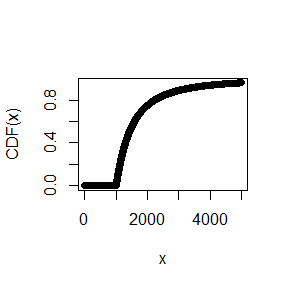dPareto(x, 1000, 2)
##   2.000000e-03 2.500000e-04 7.407407e-05 3.125000e-05 1.600000e-05
##   9.259259e-06 5.830904e-06 3.906250e-06 2.743484e-06 2.000000e-06
plot(dPareto(1:5000, 1000, 2), xlab = "x", ylab = "PDF(x)")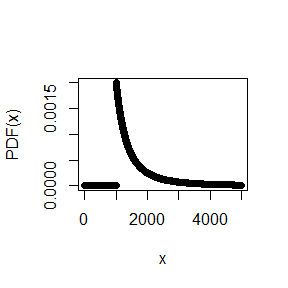The package also provides the quantile function:

qPareto(0:10 / 10, 1000, 2)
##   1000.000 1054.093 1118.034 1195.229 1290.994 1414.214 1581.139 1825.742
##   2236.068 3162.278      Inf

### Simulation:

rPareto(20, 1000, 2)
##   1361.460 1592.590 1139.756 1101.846 1019.194 5062.058 1368.522 1167.435
##   1137.132 4819.925 2563.101 1191.829 1865.089 1864.219 1340.808 1920.688
##  1307.672 1130.390 1821.301 1885.846

### Layer mean:

Let $$X\sim \text{Pareto}(t,\alpha)$$ and $$a, c\ge 0$$. Then $E(\min[c,\max(X-a,0)]) = \int_a^{c+a}(1-F_{t,\alpha}(x))\, dx =: I_{t,\alpha}^{\text{c xs a}}$ is the layer mean of $$c$$ xs $$a$$, i.e. the expected loss to the layer given a single loss $$X$$.

Example: $$t=500$$, $$\alpha = 2$$, Layer 4000 xs 1000

Pareto_Layer_Mean(4000, 1000, 2, t = 500)
##  200

### Layer variance:

Let $$X\sim \text{Pareto}(t,\alpha)$$ and $$a, c\ge 0$$. Then the variance of the layer loss $$\min[c,\max(X-a,0)]$$ can be calculated with the function Pareto_Layer_Var.

Example: $$t=500$$, $$\alpha = 2$$, Layer 4000 xs 1000

Pareto_Layer_Var(4000, 1000, 2, t = 500)
##  364719

### Pareto extrapolation

Consider two layers $$c_i$$ xs $$a_i$$ and a $$\text{Pareto}(t,\alpha)$$ distributed severity with sufficiently small $$t$$. What is the expected loss of $$c_2$$ xs $$a_2$$ given the expected loss of $$c_1$$ xs $$a_1$$?

Example: Assume $$\alpha = 2$$ and the expected loss of 4000 xs 1000 is 500. Calculate the expected loss of the layer 5000 xs 5000.

Pareto_Extrapolation(4000, 1000, 5000, 5000, 2) * 500
##  62.5
Pareto_Extrapolation(4000, 1000, 5000, 5000, 2, ExpLoss_1 = 500)
##  62.5

### Pareto alpha between two layers:

Given the expected losses of two layers, there is typically a unique Pareto alpha $$\alpha$$ which is consistent with the ratio of the expected layer losses.

Example: Expected loss of 4000 xs 1000 is 500. Expected loss of 5000 xs 5000 is 62.5. Alpha between the two layers:

Pareto_Find_Alpha_btw_Layers(4000, 1000, 500, 5000, 5000, 62.5)
##  2

Check: see previous example

### Pareto alpha between a frequency and layer:

Given the expected excess frequency at a threshold and the expected loss of a layer, then there is typically a unique Pareto alpha $$\alpha$$ which is consistent with this data.

Example: Expected frequency in excess of 500 is 2.5. Expected loss of 4000 xs 1000 is 500. Alpha between the frequency and the layer:

Pareto_Find_Alpha_btw_FQ_Layer(500, 2.5, 4000, 1000, 500)
##  2

Check:

Pareto_Layer_Mean(4000, 1000, 2, t = 500) * 2.5
##  500

### Matching the expected losses of two layers:

Given the expected losses of two layers, we can use these techniques to obtain a Poisson-Pareto model which matches the expected loss of both layers.

Example: Expected loss of 30 xs 10 is 26.66 (Burning Cost). Expected loss of 60 xs 40 is 15.95 (Exposure model).

Pareto_Find_Alpha_btw_Layers(30, 10, 26.66, 60, 40, 15.95)
##  1.086263

Frequency @ 10:

26.66 / Pareto_Layer_Mean(30, 10, 1.086263)
##  2.040392

A collective model $$\sum_{n=1}^NX_n$$ with $$X_N\sim \text{Pareto}(10, 1.09)$$ and $$N\sim \text{Poisson}(2.04)$$ matches both expected layer losses.

### Frequency extrapolation and alpha between frequencies:

Given the frequency $$f_1$$ in excess of $$t_1$$ the frequency $$f_2$$ in excess of $$t_2$$ can directly be calculated as follows: $f_2 = f_1 \cdot \left(\frac{t_1}{t_2}\right)^\alpha$ Vice versa, we can calculate the Pareto alpha, if the two excess frequencies $$f_1$$ and $$f_2$$ are given: $\alpha = \frac{\log(f_2/f_1)}{\log(t_1/t_2)}.$

Example:

Expected frequency excess 1000 is 2. What is the expected frequency excess 4000 if we have a Pareto alpha of 2.5?

t_1 <- 1000
f_1 <- 2
t_2 <- 4000
(f_2 <- f_1 * (t_1 / t_2)^2.5)
##  0.0625

Vice versa:

Pareto_Find_Alpha_btw_FQs(t_1, f_1, t_2, f_2)
##  2.5

### Maximum likelihood estimation of the parameter alpha

For $$i=1,\dots,n$$ let $$X_i\sim \text{Pareto}(t,\alpha)$$ be Pareto distributed observations. Then we have the ML estimator $\hat{\alpha}^{ML}=\frac{n}{\sum_{i=1}^n\log(X_i/t)}.$ Example:

Pareto distributed losses with a reporting threshold of $$t=1000$$ and $$\alpha = 2$$:

losses <- rPareto(1000, t = 1000, alpha = 2)
Pareto_ML_Estimator_Alpha(losses, t = 1000)
##  2.088758

In reinsurance, sometimes large loss data from different sources are used for severity fits. Then the losses are typically only available in excess of certain reporting thresholds which may vary by data source. Assume that two data sources each contain 5000 losses in excess of 1000, which are Pareto distributed with an alpha of 2 but from data source 2 we only know the losses exceeding a reporting threshold of 3000. If we apply the standard ML estimator with a threshold of 1000, then we obtain an alpha which is too low, since we ignore that the loss data is not complete in excess of 1000:

losses_1 <- rPareto(5000, t = 1000, alpha = 2)
losses_2 <- rPareto(5000, t = 1000, alpha = 2)
reported <- losses_2 > 3000
losses_2 <- losses_2[reported]
losses <- c(losses_1, losses_2)
Pareto_ML_Estimator_Alpha(losses, t = 1000)
##  1.632439

In the function Pareto_ML_Estimator_Alpha the user can define reporting threshold for each loss in order to handle this situation:

reporting_thresholds_1 <- rep(1000, length(losses_1))
reporting_thresholds_2 <- rep(3000, length(losses_2))
reporting_thresholds <- c(reporting_thresholds_1, reporting_thresholds_2)
Pareto_ML_Estimator_Alpha(losses, t = 1000, reporting_thresholds = reporting_thresholds)
##  2.011448

Now, assume that the underlying policies have limits of 5000 or 10000 and that a loss is censored if it exceeds the respective limit. If the underlying losses are Pareto distributed before they are censored then ML estimation leads to a too large value for alpha:

limits <- sample(c(5000, 10000), length(losses), replace = T)
censored <- losses > limits
losses[censored] <- limits[censored]
reported <- losses > reporting_thresholds
losses <- losses[reported]
reporting_thresholds <- reporting_thresholds[reported]
Pareto_ML_Estimator_Alpha(losses, t = 1000, reporting_thresholds = reporting_thresholds)
##  2.114551

In order to deal with this situation the function allows to specify for each loss if it is censored or not:

Pareto_ML_Estimator_Alpha(losses, t = 1000, reporting_thresholds = reporting_thresholds,
is.censored = censored)
##  2.017282

### Truncation

Let $$X\sim \text{Pareto}(t,\alpha)$$ and $$T>t$$. Then $$X|(X<T)$$ has a truncated Pareto distribution. The Pareto functions mentioned above are also available for the truncated Pareto distribution.

## Piecewise Pareto distribution

Definition: Let $$\mathbf{t}:=(t_1,\dots,t_n)$$ be a vector of thresholds with $$0<t_1<\dots<t_n<t_{n+1}:=+\infty$$ and let $$\boldsymbol\alpha:=(\alpha_1,\dots,\alpha_n)$$ be a vector of Pareto alphas with $$\alpha_i\ge 0$$ and $$\alpha_n>0$$. The piecewise Pareto distribution} $$\text{PPareto}(\mathbf{t},\boldsymbol\alpha)$$ is defined by the distribution function $F_{\mathbf{t},\boldsymbol\alpha}(x):=\begin{cases} 0 & \text{ for x<t_1} \\ \displaystyle 1-\left(\frac{t_{k}}{x}\right)^{\alpha_k}\prod_{i=1}^{k-1}\left(\frac{t_i}{t_{i+1}}\right)^{\alpha_i} & \text{ for x\in [t_k,t_{k+1}).} \end{cases}$

The family of piecewise Pareto distributions is very flexible:

Proposition: The set of Piecewise Pareto distributions is dense in the space of all positive-valued distributions (with respect to the Lévy metric).

This means that we can approximate any positive valued distribution as good as we want with piecewise Pareto. A very good approximation typically comes at the cost of many Pareto pieces. Piecewise Pareto is often a good alternative to a discrete distribution, since it is much better to handle!

The Pareto package also provides functions for the piecewise Pareto distribution. For instance:

### Distribution function

x <- c(1:10) * 1000
t <- c(1000, 2000, 3000, 4000)
alpha <- c(2, 1, 3, 20)
pPiecewisePareto(x, t, alpha)
##   0.0000000 0.7500000 0.8333333 0.9296875 0.9991894 0.9999789 0.9999990
##   0.9999999 1.0000000 1.0000000
plot(pPiecewisePareto(1:5000, t, alpha), xlab = "x", ylab = "CDF(x)")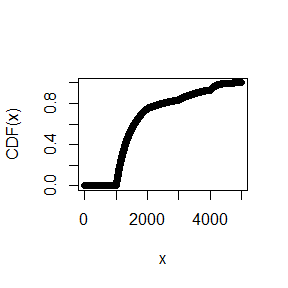### Density

dPiecewisePareto(x, t, alpha)
##   2.000000e-03 1.250000e-04 1.666667e-04 3.515625e-04 3.242592e-06
##   7.048328e-08 2.768239e-09 1.676381e-10 1.413089e-11 1.546188e-12
plot(dPiecewisePareto(1:5000, t, alpha), xlab = "x", ylab = "PDF(x)")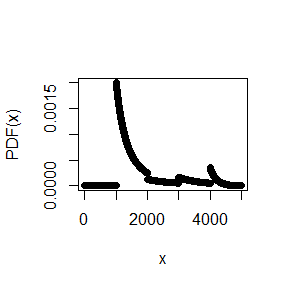### Simulation

rPiecewisePareto(20, t, alpha)
##   1063.613 1002.612 1669.623 1846.692 1496.333 2879.062 2880.773 1103.929
##   1068.508 1255.879 1310.318 1703.050 1286.462 3796.485 1181.393 1975.146
##  4036.377 1570.224 2922.042 3422.376

### Layer mean

PiecewisePareto_Layer_Mean(4000, 1000, t, alpha)
##  826.6969

### Layer variance

PiecewisePareto_Layer_Var(4000, 1000, t, alpha)
##  922221.2

### Maximum likelihood estimation of the alphas

Let $$\mathbf{t}:=(t_1,\dots,t_n)$$ be a vector of thresholds and let $$\boldsymbol\alpha:=(\alpha_1,\dots,\alpha_n)$$ be a vector of Pareto alphas. For $$i=1,\dots,n$$ let $$X_i\sim \text{PPareto}(\mathbf{t},\boldsymbol\alpha)$$. If the vector $$\mathbf{t}$$ is known, then the parameter vector $$\boldsymbol\alpha$$ can be estimated with maximum likelihood.

Example:

Piecewise Pareto distributed losses with $$\mathbf{t}:=(1000,\,2000,\, 3000)$$ and $$\boldsymbol\alpha:=(1,\, 2,\, 3)$$:

losses <- rPiecewisePareto(10000, t = c(1000, 2000, 3000), alpha = c(1, 2, 3))
PiecewisePareto_ML_Estimator_Alpha(losses, c(1000, 2000, 3000))
##  1.011585 1.999219 2.965304

Reporting thresholds and censoring of losses can be taken into account as described for the function Pareto_ML_Estimator_Alpha.

losses_1 <- rPiecewisePareto(5000, t = c(1000, 2000, 3000), alpha = c(1, 2, 3))
losses_2 <- rPiecewisePareto(5000, t = c(1000, 2000, 3000), alpha = c(1, 2, 3))
reported <- losses_2 > 3000
losses_2 <- losses_2[reported]
losses <- c(losses_1, losses_2)
PiecewisePareto_ML_Estimator_Alpha(losses, c(1000, 2000, 3000))
##  0.7834893 1.2457727 2.9783761
reporting_thresholds_1 <- rep(1000, length(losses_1))
reporting_thresholds_2 <- rep(3000, length(losses_2))
reporting_thresholds <- c(reporting_thresholds_1, reporting_thresholds_2)
PiecewisePareto_ML_Estimator_Alpha(losses, c(1000, 2000, 3000),
reporting_thresholds = reporting_thresholds)
##  1.022747 2.068119 2.978376
limits <- sample(c(2500, 5000, 10000), length(losses), replace = T)
censored <- losses > limits
losses[censored] <- limits[censored]
reported <- losses > reporting_thresholds
losses <- losses[reported]
reporting_thresholds <- reporting_thresholds[reported]
censored <- censored[reported]
PiecewisePareto_ML_Estimator_Alpha(losses, c(1000, 2000, 3000),
reporting_thresholds = reporting_thresholds)
##  1.022747 2.963881 3.388210
PiecewisePareto_ML_Estimator_Alpha(losses, c(1000, 2000, 3000),
reporting_thresholds = reporting_thresholds,
is.censored = censored)
##  1.022747 2.080627 2.957848

### Truncation

The package also provides truncated versions of the piecewise Pareto distribution. There are two options available:

• truncation_type = 'lp': Below the largest threshold $$t_n$$, the distribution function equals the distribution of the piecewise Pareto distribution without truncation. The last Pareto piece, however, is truncated at truncation
• truncation_type = 'wd': The whole piecewise Pareto distribution is truncated at truncation’

## Matching a tower of layer losses

The Pareto distribution can be used to build a collective model which matches the expected loss of two layers. We can use piecewise Pareto if we want to match the expected loss of more than two layers.

Consider a sequence of attachment points $$0 < a_1 <\dots < a_n<a_{n+1}:=+\infty$$. Let $$c_i:=a_{i+1}-a_i$$ and let $$e_i$$ be the expected loss of the layer $$c_i$$ xs $$a_i$$. Moreover, let $$f_1$$ be the expected frequency in excess of $$a_1$$.

The following matching algorithm uses one Pareto piece per layer and is straight forward:

• Calculate the Pareto alpha $$\alpha_1$$ between the excess frequency $$f_1$$ and the layer $$c_1$$ xs $$a_1$$
• Calculate the frequency $$f_2$$ in excess of $$a_2$$: $$f_2:=(a_1/a_2)^{\alpha_1}\cdot f_1$$
• Calculate the Pareto alpha $$\alpha_2$$ between the excess frequency $$f_2$$ and the layer $$c_2$$ xs $$a_2$$
• Calculate the frequency $$f_3$$ in excess of $$a_3$$: $$f_3:=(a_2/a_3)^{\alpha_2}\cdot f_3$$
• $$\dots$$
• Use a collective model $$\sum_{n=1}^NX_n$$ with $$E(N)=f_1$$ and $$X_n\sim\text{PPareto}(\mathbf{t},\boldsymbol\alpha)$$.

This approach always works for three layers, but it often does not work if we have three or more layers. For instance, Riegel (2018) shows that it does not work for the following example:

$$i$$ Cover $$c_i$$ Att. Pt. $$a_i$$ Exp. Loss $$e_i$$ Rate on Line $$e_i/c_i$$
1 500 1000 100 0.20
2 500 1500 90 0.18
3 500 2000 50 0.10
4 500 2500 40 0.08

The Pareto package provides a more complex matching approach that uses two Pareto pieces per layer. Riegel (2018) shows that this approach works for an arbitrary number of layers with consistent expected losses.

Example:

attachment_points <- c(1000, 1500, 2000, 2500, 3000)
exp_losses <- c(100, 90, 50, 40, 100)
fit <- PiecewisePareto_Match_Layer_Losses(attachment_points, exp_losses)
fit
##
## Panjer & Piecewise Pareto model
##
## Collective model with a Poisson distribution for the claim count and a Piecewise Pareto distributed severity.
##
## Poisson Distribution:
## Expected Frequency:   0.2136971
##
## Piecewise Pareto Distribution:
## Thresholds:         1000   1500   1932.059   2000   2147.531   2500   2847.756   3000
## Alphas:              0.3091209   0.1753613   9.685189   3.538534   0.817398   0.7663698   5.086828   2.845488
## The distribution is not truncated.
##
## Status:               0
## Comments:             OK

The function PiecewisePareto_Match_Layer_Losses returns a PPP_Model object (PPP stands for Panjer & Piecewise Pareto) which contains the information required to specify a collective model with a Panjer distributed claim count and a piecewise Pareto distributed severity. The results can be checked using the attributes FQ, t and alpha of the object:

c(PiecewisePareto_Layer_Mean(500, 1000, fit$t, fit$alpha) * fit$FQ, PiecewisePareto_Layer_Mean(500, 1500, fit$t, fit$alpha) * fit$FQ,
PiecewisePareto_Layer_Mean(500, 2000, fit$t, fit$alpha) * fit$FQ, PiecewisePareto_Layer_Mean(500, 2500, fit$t, fit$alpha) * fit$FQ,
PiecewisePareto_Layer_Mean(Inf, 3000, fit$t, fit$alpha) * fit\$FQ)
##  100  90  50  40 100

There are, however, functions which can directly use PPP_Models:

covers <- c(diff(attachment_points), Inf)
Layer_Mean(fit, covers, attachment_points)
##  100  90  50  40 100

## Matching reference information

The function PiecewisePareto_Match_Layer_Losses can be used to match the expected losses of a complete tower of layers. If we want to match the expected losses of some reference layers which do not form a complete tower then it is more convenient to use the function Fit_References. Also excess frequencies can be provided as reference information. The function can be seen as a user interface for PiecewisePareto_Match_Layer_Losses:

  covers <- c(1000, 1000, 1000)
att_points <- c(1000, 2000, 5000)
exp_losses <- c(100, 50, 10)
thresholds <- c(4000, 10000)
fqs <- c(0.04, 0.005)
fit <- Fit_References(covers, att_points, exp_losses, thresholds, fqs)
Layer_Mean(fit, covers, att_points)
##  100  50  10
  Excess_Frequency(fit, thresholds)
##  0.040 0.005

If the package lpSolve is installed then the funcion Fit_References can handle ovelapping layers.

## Interpolation of PML curves

The function Fit_PML_Curve can be used fit a PPP_Model that reproduces and interpolates the information provided in the PML curve. A PML curve is a table containing return periods and the corresponding loss amounts:

$$i$$ Return Period $$r_i$$ Amount $$x_i$$
1 1 1000
2 5 4000
3 10 7000
4 20 10000
5 50 13000
6 100 14000

The information contained in such a PML curve can be used to create a PPP_Model that has the expected excess frequency $$1/r_i$$ at $$x_i$$.

Example:

return_periods <- c(1, 5, 10, 20, 50, 100)
amounts <- c(1000, 4000, 7000, 10000, 13000, 14000)
fit <- Fit_PML_Curve(return_periods, amounts)
1 / Excess_Frequency(fit, amounts)
##    1   5  10  20  50 100

## PPP_Models (Panjer & Piecewise Pareto Models)

A PPP_Model object contains the information required to specify a collective model with a Panjer distributed claim count and a piecewise Pareto distributed severity.

Claim count distribution: The Panjer class contains the binomial distribution, the Poisson distribution and the negative binomial distribution. The distribution of the claim count $$N$$ is specified by the expected frequency $$E(N)$$ (attribute FQ of the object) and the dispersion $$D(N):=Var(N)/E(N)$$ (attribute dispersion of the object). We have the following cases:

• dispersion < 1: binomial distribution
• dispersion = 1: Poisson distribution
• dispersion > 1: negative binomial distribution.

Severity distribution: The piecewise Pareto distribution is specified by the vectors t, alpha, truncation and truncation_type.

The function PiecewisePareto_Match_Layer_Losses returns PPP_Model object. Such an object can also be directly created using the constructor function:

PPPM <- PPP_Model(FQ = 2, t = c(1000, 2000), alpha = c(1, 2),
truncation = 10000, truncation_type = "wd", dispersion = 1.5)
PPPM
##
## Panjer & Piecewise Pareto model
##
## Collective model with a Negative Binomial distribution for the claim count and a Piecewise Pareto distributed severity.
##
## Negative Binomial Distribution:
## Expected Frequency:   2
## Dispersion:           1.5 (i.e. contagion = 0.25)
##
## Piecewise Pareto Distribution:
## Thresholds:         1000   2000
## Alphas:              1   2
## Truncation:           10000
## Truncation Type:      'wd'
##
## Status:               0
## Comments:             OK

### Expected Loss, Standard Deviation and Variance for Reinsurance Layers

A PPP_Model can directly be used to calculate the expected loss, the standard deviation or the variance of a reinsurance layer: function:

PPPM <- PPP_Model(FQ = 2, t = c(1000, 2000), alpha = c(1, 2),
truncation = 10000, truncation_type = "wd", dispersion = 1.5)
Layer_Mean(PPPM, 4000, 1000)
##  2475.811
Layer_Sd(PPPM, 4000, 1000)
##  2676.332
Layer_Var(PPPM, 4000, 1000)
##  7162754

### Expected Excess Frequency

A PPP_Model can directly be used to calculate the expected frequency in excess of a threshold:

PPPM <- PPP_Model(FQ = 2, t = c(1000, 2000), alpha = c(1, 2),
truncation = 10000, truncation_type = "wd", dispersion = 1.5)
thresholds <- c(0, 1000, 2000, 5000, 10000, Inf)
Excess_Frequency(PPPM, thresholds)
##  2.0000000 2.0000000 0.9795918 0.1224490 0.0000000 0.0000000

### Simulation of Losses

A PPP_Model can directly be used to simulate losses with the corresponding collective model:

PPPM <- PPP_Model(FQ = 2, t = c(1000, 2000), alpha = c(1, 2),
truncation = 10000, truncation_type = "wd", dispersion = 1.5)
Simulate_Losses(PPPM, 10)
##           [,1]     [,2]     [,3]     [,4]     [,5]
##  [1,] 2854.931      NaN      NaN      NaN      NaN
##  [2,]      NaN      NaN      NaN      NaN      NaN
##  [3,] 1724.511 1899.499 3054.764 2522.207      NaN
##  [4,] 2156.510 1290.258      NaN      NaN      NaN
##  [5,] 4159.505 1021.784 2926.003      NaN      NaN
##  [6,] 4992.884 1671.463 1155.838 1295.721 1138.447
##  [7,] 1241.865 2471.538      NaN      NaN      NaN
##  [8,]      NaN      NaN      NaN      NaN      NaN
##  [9,] 2098.202 1045.150 3903.733      NaN      NaN
## [10,] 2365.764 1757.188 1106.682      NaN      NaN

The function Simulate_Losses returns a matrix where each row contains the losses from one simulation.

Note that for a given expected frequency FQ not every dispersion dispersion < 1 is possible for the binomial distribution. In this case a binomial distribution with the smallest dispersion larger than or equal to dispersion is used for the simulation.

## Generalized Pareto Distribution

Definition: Let $$t>0$$ and $$\alpha_\text{ini}, \alpha_\text{tail}>0$$. The generalized Pareto distribution $$\text{GenPareto}(t,\alpha_\text{ini}, \alpha_\text{tail})$$ is defined by the distribution function $F_{t,\alpha_\text{ini}, \alpha_\text{tail}}(x):=\begin{cases} 0 & \text{ for x\le t} \\ \displaystyle 1-\left(1+\frac{\alpha_\text{ini}}{\alpha_\text{tail}} \left(\frac{x}{t}-1\right)\right)^{-\alpha_\text{tail}} & \text{ for x>t.} \end{cases}$ We do not the standard parameterization from extreme value theory but the parameterization from Riegel (2008) which is useful in a reinsurance context.

### Distribution function and density

The functions pGenPareto and dGenPareto provide the distribution function and the density function of the Pareto distribution:

x <- c(1:10) * 1000
pGenPareto(x, t = 1000, alpha_ini = 1, alpha_tail = 2)
##   0.0000000 0.5555556 0.7500000 0.8400000 0.8888889 0.9183673 0.9375000
##   0.9506173 0.9600000 0.9669421
plot(pGenPareto(1:5000, 1000, 1, 2), xlab = "x", ylab = "CDF(x)")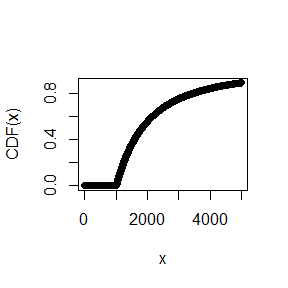dGenPareto(x, t = 1000, alpha_ini = 1, alpha_tail = 2)
##   1.000000e-03 2.962963e-04 1.250000e-04 6.400000e-05 3.703704e-05
##   2.332362e-05 1.562500e-05 1.097394e-05 8.000000e-06 6.010518e-06
plot(dGenPareto(1:5000, 1000, 1, 2), xlab = "x", ylab = "PDF(x)")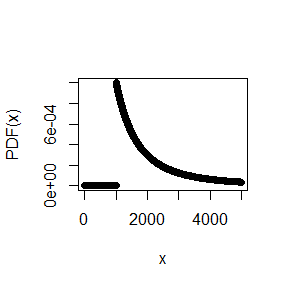The package also provides the quantile function:

qGenPareto(0:10 / 10, 1000, 1, 2)
##   1000.000 1108.185 1236.068 1390.457 1581.989 1828.427 2162.278 2651.484
##   3472.136 5324.555      Inf

### Simulation:

rGenPareto(20, 1000, 1, 2)
##    3250.889  1838.973  6065.089  1671.509  1703.054 16647.045  1254.244
##    1169.038  1127.066  1241.358  4028.926  1085.437  1747.497  2472.309
##   1426.704  1002.570  1304.151  1412.421  5947.293  4410.795

### Layer mean:

GenPareto_Layer_Mean(4000, 1000, t = 500, alpha_ini = 1, alpha_tail = 2)
##  484.8485

### Layer variance:

GenPareto_Layer_Var(4000, 1000, t = 500, alpha_ini = 1, alpha_tail = 2)
##  908942.5

### Maximum likelihood estimation of the alpha_ini and alpha_tail

Let $$t>0$$ and $$\alpha_\text{ini}, \alpha_\text{tail}>0$$ and let $$X_i\sim \text{GenPareto}(t,\alpha_\text{ini}, \alpha_\text{tail})$$. For known $$t$$ the parameters $$\alpha_\text{ini}, \alpha_\text{tail}$$ can be estimated with maximum likelihood.

Example:

Generalized Pareto distributed losses with $$t:=1000$$ and $$\alpha_\text{ini}=1$$, $$\alpha_\text{tail}=2$$:

losses <- rGenPareto(10000, t = 1000, alpha_ini = 1, alpha_tail = 2)
GenPareto_ML_Estimator_Alpha(losses, 1000)
##  0.9787852 2.0844113

Reporting thresholds and censoring of losses can be taken into account as described for the function Pareto_ML_Estimator_Alpha.

losses_1 <- rGenPareto(5000, t = 1000, alpha_ini = 1, alpha_tail = 2)
losses_2 <- rGenPareto(5000, t = 1000, alpha_ini = 1, alpha_tail = 2)
reported <- losses_2 > 3000
losses_2 <- losses_2[reported]
losses <- c(losses_1, losses_2)
GenPareto_ML_Estimator_Alpha(losses, 1000)
##  0.635907 2.197439
reporting_thresholds_1 <- rep(1000, length(losses_1))
reporting_thresholds_2 <- rep(3000, length(losses_2))
reporting_thresholds <- c(reporting_thresholds_1, reporting_thresholds_2)
GenPareto_ML_Estimator_Alpha(losses, 1000,
reporting_thresholds = reporting_thresholds)
##  0.9576829 1.9998593
limits <- sample(c(2500, 5000, 10000), length(losses), replace = T)
censored <- losses > limits
losses[censored] <- limits[censored]
reported <- losses > reporting_thresholds
losses <- losses[reported]
reporting_thresholds <- reporting_thresholds[reported]
censored <- censored[reported]
GenPareto_ML_Estimator_Alpha(losses, 1000,
reporting_thresholds = reporting_thresholds)
##  0.8887291 5.9222437
GenPareto_ML_Estimator_Alpha(losses, 1000,
reporting_thresholds = reporting_thresholds,
is.censored = censored)
##  0.963454 1.962438

### Truncation

Let $$X\sim \text{GenPareto}(t, \alpha_\text{ini}, \alpha_\text{tail})$$ and $$T>t$$. Then $$X|(X<T)$$ has a truncated generalized Pareto distribution. The Pareto functions mentioned above are also available for the truncated generalized Pareto distribution.

## PGP_Models (Panjer & Generalized Pareto Models)

A PGP_Model object contains the information required to specify a collective model with a Panjer distributed claim count and a generalized Pareto distributed severity.

Claim count distribution: Like in a PPP_Model the claim count distribution from the Panjer class is specified by the expected frequency $$E(N)$$ (attribute FQ of the object) and the dispersion $$D(N):=Var(N)/E(N)$$ (attribute dispersion of the object).

Severity distribution: The generalized Pareto distribution is specified by the parameters t, alpha_ini, alpha_tail and truncation.

A PPP_Model object can be created using the constructor function:

PGPM <- PGP_Model(FQ = 2, t = 1000, alpha_ini = 1, alpha_tail = 2,
truncation = 10000, dispersion = 1.5)
PGPM
##
## Panjer & Generalized Pareto model
##
## Collective model with a Negative Binomial distribution for the claim count and a generalized Pareto distributed severity.
##
## Negative Binomial Distribution:
## Expected Frequency:   2
## Dispersion:           1.5 (i.e. contagion = 0.25)
## Generalized Pareto Distribution:
## Threshold:            1000
## alpha_ini:            1
## alpha_tail:           2
## Truncation:           10000
##
## Status:               0
## Comments:             OK

### Methods for PGP_Models

For PGP_Models the same methods are available as for PPP_Models:

PGPM <- PGP_Model(FQ = 2, t = 1000, alpha_ini = 1, alpha_tail = 2,
truncation = 10000, dispersion = 1.5)
Layer_Mean(PGPM, 4000, 1000)
##  2484.33
Layer_Sd(PGPM, 4000, 1000)
##  2756.15
Layer_Var(PGPM, 4000, 1000)
##  7596365
thresholds <- c(0, 1000, 2000, 5000, 10000, Inf)
Excess_Frequency(PGPM, thresholds)
##  2.0000000 2.0000000 0.8509022 0.1614435 0.0000000 0.0000000
Simulate_Losses(PGPM, 10)
##           [,1]     [,2]     [,3]     [,4]
##  [1,] 1521.848 1194.747 2172.534      NaN
##  [2,] 1361.549 4106.740      NaN      NaN
##  [3,] 3299.299 1553.965      NaN      NaN
##  [4,] 2635.675 1036.588 1096.835      NaN
##  [5,] 3734.274      NaN      NaN      NaN
##  [6,] 3185.860 4633.497 4676.543 1047.863
##  [7,] 5123.614 2463.131 1157.193      NaN
##  [8,] 2265.114 1080.051 4129.473      NaN
##  [9,] 1066.235 2384.368      NaN      NaN
## [10,]      NaN      NaN      NaN      NaN`